Amsat-UK's Oscar News. In 3 parts:
1. 1984 Dec, No.50 p.2-6
2. 1985 Feb, No.51 p.2-6
3. 1985 Apr, No.52 p.8-11

# SUN'S UP!

### by James Miller G3RUH

```Historical Note
---------------
This was my first "serious" article about astro-numerical stuff.  It was
written at a time when few people had computers, and even fewer knew
how to use them.  So to some extent the material was aimed as being do-able
on a hand calculator as well.  Again, at the time there was a total dearth
of technical matter as herein described, although I didn't know so.  I was
simply pouring it out as a kind of public service.   On reflection it's a
bit clumsy and today I'd do it differently, but I hope it's still useful.

This was also the series that got me "noticed" by the then AO-10 command
stations.  I was invited to generate input to help plan attitude schedules
for the satellite.  This I did, and also wrote a lot of navigation
software which is still in daily use (1994) with Oscar-13.          JRM
---------------------------------------------------------------------------

SUN'S UP!  Part 1

by J.R. Miller G3RUH

Introduction
------------
A grand numerical offering this month - but don't panic,  self-training
does not lead to blindness!

In the last issue I said that I'd write about modelling the Sun's orbit,
so here it is.  This information can be used to predict
eclipses/shadowing, to check spacecraft illumination, computerise an
alamanac, to navigate or simply to calibrate your garden sun dial.

I had hoped to include some applications this session, but the space-time
warp got me so it's carried forward to the next issue.  Then I'll include
calculating the angle of the Sun above or below the Oscar-10 orbit
plane, and the vital solar panel illumination coefficient.  If there's
still space I'll then show how shadowing (eclipse) is predicted.

Most of these ideas are embodied in an updated version of the program
OSCAR10.BAS which appeared in O.N. 45 (Dec 1983), renamed PLAN10 and coded
now for the BBC computer.  This is shortly to be on sale via AMSAT, as is
a listing for conversion into other BASIC dialects.  [Updated 1990 Oct].

Sun Model
---------
The Sun revolves around  the  Earth (sorry Copernicus but it's all
relative, mate) just like any other satellite.  So finding its position is
just as easy!  Looking further down this spiel may look horrendous, but
experience shows that full frontal brevity results in mass confusion and
a flood of mail, so I've tried to include everything.

The Sun has  an  almost  circular  orbit, e  =  0.017, and the time
between  equator  crossings, called a tropical year, is 365.2422 days.
Perigee (perihelion) is slowly moving so the time between successve
perigees or anomalistic period is 365.2596 days.  The orbit plane
(ecliptic) is inclined at 23.44 degrees to the equatorial plane.

The Sun's ascending  node DEFINES the celestial origin, (the First Point
in Aries).  Thus the Sun's  RAAN is always zero.  To complete the
ephemeris we need a reference time (epoch), a position round the orbit
(mean anomaly), and  the perigee position (or equivalent) at that time.

First I give the Sun's ephemeris  for epoch 1990 Jan 0.0.  To find the
Sun's location at any other time first calculate the elapsed time since
epoch (step 1).  In step 2 mean anomaly etc are cranked on to the time of
interest.  What you do next depends on what answers you want.

Step 3a gives basic rectangular coordinates, such as you might use for
satellite illumination studies.  It's also the stepping stone to the other
coordinates.  Step 3b converts to an Earth based frame which will yield
lat/long if required.  Step 4a produces the astronomic coordinates
(declination  and right ascension) which can also be reduced to lat/lon
(step 4b).  The equation of time is appended in step 4c.

GHAA (Greenwich hour angle Aries) is the same quantity that also features
in most satellite position calculations.

IMPORTANT: Now Read This
------------------------
1. All angles are in DEGREES, and if your calculator/computer works in
radians then take appropriate measures.

2. INT(X)  means take the integer part of X, and is defined as the integer
just LESS than X.  Thus INT(-1.6) is -2.  Some machines will return -1, so
take care.  This definition is regular through zero. ATN means arctangent,
ASN means arcsine.  ASN(X) = ATN(X/(SQR(1-X*X)). SQR means square root.

3. In the following equations it is implicit that any angle A is in the
range 0 <= A < 360 degrees.  Before re-use any result should be so bounded
if need be.  Effect this by A = 360*(A-INT(A/360)).  Failure to heed this
suggestion may result in clumsy answers.

4. The coordinate transformations are obviously interlinked, and not
unique.  Rearrange them as you will.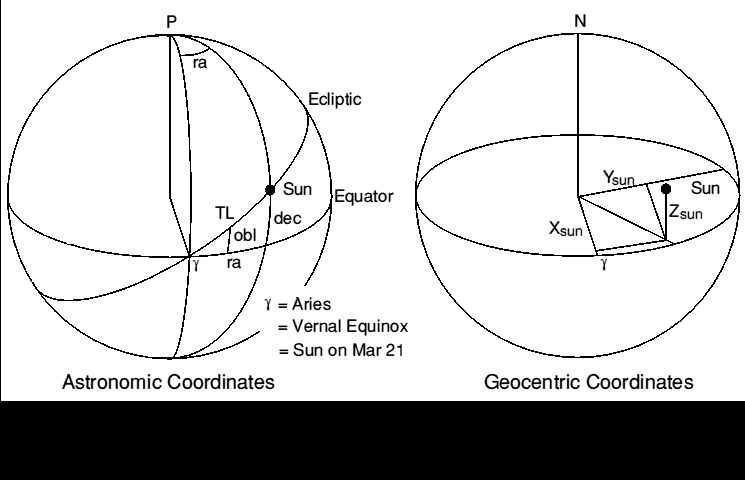Figure 0. Showing astronomic and geocentric coordinate systems.

Solar Ephemeris at 1990 Jan 0.0
-------------------------------
DAY0     726482         Day number of Epoch (origin approx. year BC 1)
M0      356.6349        Mean anomaly at epoch
L0      279.4033        Mean longitude along ecliptic
EQC1      1.9151        Equation of centre harmonic coefficient
EQC2      0.0200                "       "       "       "
OBLQ     23.4406        Obliquity of ecliptic (inclination)

Step 1. Calculate number of days since epoch
--------------------------------------------
Enter Year (e.g.1985), Day number (Jan 1 = 1), hours, minutes, seconds:

YRDAY =   INT( (YEAR-1)*365.25 ) - DAY0         Days from year difference
FRDAY =  ( HOUR + ( MIN + SEC/60 )/60 )/24      Fraction of day
TIM   =   YRDAY + DAYNO + FRDAY                 Time in days since epoch

Note: These formulae only valid 1900 Mar 01 - 2100 Feb 28.

Step 2. Compute GHAA and True Longitude
---------------------------------------
MA   = M0   +   360 * TIM / 365.2596413         Sun's Mean Anomaly
ML   = L0   +   360 * TIM / 365.2421988         Mean Longitude
GHAA = ML-180 + 360 * FRDAY                     Greenwich Hour Angle Aries

TL   = ML + EQC1*SIN(MA) + EQC2*SIN(2*MA)       True Longitude

Note:   1. 'Longitude' means angle from ascending node up the ecliptic.
2. The Sun's argument of perigee (AP) is given by  AP = ML - MA

Step 3a. Compute Unit Vector, Geocentric Rectangular Coordinates
----------------------------------------------------------------
Xsun = COS(TL)                                  ( =  COS(DEC) * COS(RA) )
Ysun = SIN(TL) * COS(OBLQ)                      ( =  COS(DEC) * SIN(RA) )
Zsun = SIN(TL) * SIN(OBLQ)                      ( =  SIN(DEC)           )

Coordinate set is right-handed orthogonal located at the Earth's centre, X
and Y axes in the equatorial plane; X points at the ascending node, or the
first point in Aries (Vernal Equinox), and Y axis is at RA = 90 degrees;
Z axis normal to the plane, pointing approximately at the Pole Star.

Step 3b. Unit Vector, Geocentric, Greenwich Coordinates
-------------------------------------------------------
C  =  COS(GHAA): S= SIN(GHAA)
Xg =  C * Xsun  +  S * Ysun                     ( =  COS(LAT) * COS(-LON) )
Yg = -S * Xsun  +  C * Ysun                     ( =  COS(LAT) * SIN(-LON) )
Zg =      Zsun                                  ( =  SIN(LAT)             )

Coordinate set is right-handed orthogonal located at the Earth's centre, X
and Y axes in the equatorial plane.  X points at intersection of Greenwich
meridian and Equator, and Y axis points at longitude 90 degrees East.  Z
axis normal to the plane, pointing up through North Pole.  This set is as
the set above but rotated about the Z axis RAAN degrees East.

Step 4a.  Astronomic Coordinates
--------------------------------
DEC = ASN (Zsun)                                Declination
RA  = ATN (Ysun/Xsun)                           Right Ascension
if Xsun < 0 then RA = RA + 180                correct quadrant
if  RA  < 0 then RA = RA + 360

ATN is assumed to yield a value in the range +- 90 degrees.  RA is in the
same quadrant as true longitude, TL.

Step 4b. Geographic Coordinates
-------------------------------
LAT  = DEC                                      Sun's Latitude,  +North
LON  = GHAA - RA                                Sun's Longitude, +West

Step 4c. Equation of Time
-------------------------
EQOT = ( ML - RA ) * 4                          Minutes

Equation of time is the amount to be subtracted from 'sundial' time to
give local time, maxima being  -(14m 16s) around Feb 12th, and +(16m 24s)
around Nov 3rd.  The Sun is at maximum elevation at local noon minus EQOT.

Test Data
---------
The following example data are provided to help you to check your own
calculations.  EXAMPLE: Evaluate all data for, Aug 12th 1985 at 0145:00
GMT.  All angles reduced to range 0 - 360 degrees. From diary: DAYNO = 224

YRDAY = -1826:          FRDAY = 0.072917:       TIM = -1601.927083 days

MA   217.7751:          ML   140.4681:          TL  139.3144
GHAA 346.7181:          RA   141.7354:          DEC  15.0302

Xsun  -0.758298:        Xg    -0.875425:        LAT  15.0302 North
Ysun   0.598108:        Yg     0.407897:        LON 204.9828 West
Zsun    0.259328:       Zg     0.259328:        EQOT -(5m 4s)

Sources and Accuracy
--------------------
The solar data has been derived from:

1. The Astronomical Ephemeris 1984. ISBN:0-11-886919-1, HMSO & USGPO.

2. Explanatory Supplement to the Astronomical Ephemeris. 1961 Rev. 1974.
ISBN: 0-11-880578-9, HMSO (presently out of print, new edn. in prep.)

Angular errors are unlikely to exceed 0.2' of arc.  The data may be used
for a decade forward  or  backwards from 1990 with  graceful  degradation.
These equations describe a 'mean' Sun's motion. Discrepancies compared
with an almanac, are created partly by planetary purturbations to the
Earth's orbit, partly by equating Universal time with Ephemeris time and
partly to numerical adjustments deliberately embodied in almanac tables.
It's the Earth/Moon centre of  gravity and not the Earth's centre which
orbits the Sun.  There are other sources too - consult the references.

SUN'S UP!  Part 2

by J.R. Miller G3RUH

on the general theme of the relationship between the Sun and an Earth
satellite.  I'm using Oscar-10 in particular, but the methods are in
general applicable to any of the others.

First a few notes about vectors and then how to calculate solar elevation
above the orbit plane, and the solar panel illumination coefficient.
Eclipses will be dealt with in part 3.

Vectors
-------
Before proceeding I think it would be useful to introduce a few things
about vectors.  A vector is a neat way of describing a point or a line in
space, and vectors can be added, subtracted and multiplied, as can
ordinary numbers which are merely one-dimensional vectors.

In our context a vector consists of three numbers, representing
coordinates along the three axes.  For example a point 1 unit along the
X-axis, and zero units along the  Y  and  Z  axes can be compactly written
< 1, 0, 0>.

A line at 45 degrees to the X and Y axes, and in the same plane is
< 0.71, 0.71, 0>

A line equidistant from all three axes is
< 0.58, 0.58, 0.58>, and so on. (Sketch them).

The length of a vector < X, Y, Z > is given by  L = SQR( X*X + Y*Y + Z*Z ).
So you will see that all the above vectors are of length 1.0, and are not
suprisingly called Unit Vectors.

The angle between two unit vectors is found by what is called their scalar
(dot)  product.  Denoting unit vectors A and B by < XA, YA, ZA > and
< XB, YB, ZB> then their included angle ANG is found from:

Cos(ANG) = XA*XB + YA*YB + ZA*ZB     = < A > dot < B > = A.B

The length of vector A projected onto unit vector B (or vice versa) is
also given by their scalar product, though in this case A need not
necessarily be of unit length. This is called 'resolving A onto B'.

If the scalar product of two unit vectors is unity, then the vectors are
coincident.  If the scalar product of two vectors is zero then they are
at right angles (90 deg) to each other.  Vectors also very obligingly obey
some familiar rules  A+B = B+A, and A.B = B.A     PROVIDED they are
expressed in the same coordinate system.

Verify these easy concepts for yourself using the three unit vectors
above,  and inventing some  of  your  own.  For further reading try
"Advanced Engineering Mathematics", E. Kreyszig, John Wiley and Sons, ISBN
0-471-88941-5 or almost any similar title.

Solar Elevation above Oscar-10's Orbit
--------------------------------------
As a first example of using the sun's position  let us work out the
elevation of the Sun above (or below) Oscar-10's orbit plane.

To do this we need a vector to describe the Sun's position, and another
vector to represent the satellite's orbital plane; a perpendicular to the
plane provides just this.

Then the scalar (dot) product of these two gives the cosine of the angle
'twixt them.  We want the angle above the plane which is 90 minus this
(sketch it), i.e. the arcsine of their scalar product.

Denoting the orbit normal components by < Xnorm, Ynorm, Znorm >, the Sun's
vector as before by < Xsun, Ysun, Zsun >, and the solar elevation by SEL
we have:

SEL = ASN( Xsun*Xnorm + Ysun*Ynorm + Zsun*Znorm )

where for a plane, given its RAAN and inclination INCL the normal in
celestial coordinates is:

Xnorm =   SIN(INCL) * SIN(RAAN)
Ynorm = - SIN(INCL) * COS(RAAN)
Znorm =   COS(INCL)

(Verify this for yourself with some sketches, trying various values for
INCL and RAAN.  For example if INCL = 0 then the normal vector becomes
< 0,0,1 >, ie straight up the Z-axis, and so on).

Continuing the example (Pt.1) for 1985 Aug 12th 0145:00 GMT, DATIM =
224.072917, Oscar-10 INCL = 25.6 deg and   RAAN = 159.8 - 0.1724 * DATIM =
121.2 deg, we find:

Xnorm = 0.3696          Xsun = -0.7583          norm . Sun
Ynorm = 0.2238          Ysun =  0.5981           = 0.0874
Znorm = 0.9018          Zsun =  0.2593

and hence SEL = ASN(0.0874) = +5 deg.

(Fig. 1) When solar elevation approaches zero, as it does  twice a year,
the sun lies in the satellite orbital plane, and hence Oscar-10 passes
through the earth's shadow on each orbit.  Also the smallest angular
diameter of the Earth, seen from maximum range is +-ATN(6378/41000) = +-9
deg, so whenever the solar elevation is less than this (as in the above
example) an eclipse is inevitable at some time round the orbit.

You can also see that in 1987 the value hovers around zero for most of the
year, so we can expect some frequent changes to the operating schedule in
consequence.Figure 1. OSCAR-10  SOLAR ELEVATION 1984-7.  The maximum possible  value
is the sum of Oscar-10 and the Sun's inclinations, i.e. 25.6 + 23.4 = 49 deg.

Illumination Coefficient
------------------------
From the satellite's point of view, perhaps the single most important
parameter is the solar panel illumination coefficient, for without
sunlight a non-nuclear powered spacecraft dies.

You will know from the pictures how Oscar-10's panels are arranged, and as
the satellite spins at 30 r.p.m. or so about the axis of symmetry each
panel is illuminated in turn.

Obviously for maximum effect the Sun needs to be perpendicular to the spin
axis, whilst if it becomes co-axial then there will be no illumination.
So to analyse this problem we need a vector representing the satellite's
attitude.

If we assume that Oscar-10 is nominally oriented, then the spin axis is
exactly parallel to the orbit semi major axis (pointing in the apogee to
perigee direction), so this gives us our attitude vector.

And, as before, the cosine of the angle between Sun and spin axis is given
by the scalar product of their two unit vectors.  If we call this the Sun
Angle, then 0 deg = no illumination, 90 deg = 100% illumination.

We already have the Sun's vector, in celestial coordinates, so it remains
to translate a unit length along the spin axis into the same coordinate
set by rotating it through the various angles that define the orbit
attitude; argument of perigee (AP), inclination (INCL) and right ascension
of ascending node (RAAN).  All elliptic orbit calculation programs do
this, though probably a bit obscured.  The answer fully worked out is:

Xa =  Cos(AP) * Cos(RAAN)   -   Sin(AP) * Cos(INCL) * Sin(RAAN)
Ya =  Cos(AP) * Sin(RAAN)   +   Sin(AP) * Cos(INCL) * Cos(RAAN)
Za =                            Sin(AP) * Sin(INCL)

The sun angle (SA) and illumination coefficient (ILL) are thus:

SA    =  ACS( Xa*Xsun + Ya*Ysun + Za*Zsun)
ILL   =  100 * SIN( SA )                        (percent)

Worked example: Continuing as before for 1985 August 12 @ 0145 GMT.  First
work out argument of perigee for DATIM = 224.072917:

AP = 336.6 + 0.2834*DATIM = 400.1 = 40.1 deg (see O.N. 50), and we already
have RAAN = 121.2 and INCL = 25.6 from above.  Evaluating we find

Xa = -0.8931    Xsun = -0.7583          a . sun = 0.9608
Ya =  0.3534    Ysun =  0.5981          SA = ACS(0.9608) = 16 deg
Za =  0.2783    Zsun =  0.2593          ILL = 28%

A  value of SA in the range 0 to 90 means that the Sun is illuminating
somwhere between the spacecraft's  top and  midriff,  while  a value
90-180 degrees, the lower half.  Exactly 90 degrees is the ideal
condition, side-on.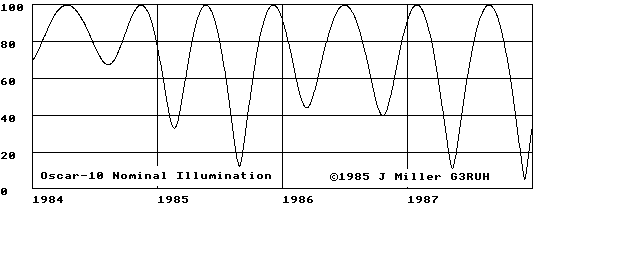Figure 2. OSCAR-10 NOMINAL ILLUMINATION computed as above for 1984 to 1987.

An illumination factor of 28% is  unacceptably low, and the managers will
have had to take corrective action long before this happens,  by
reorienting the spacecraft.

Now it is clearly desirable to keep the antennae pointing at the Earth as
far as possible, so the favoured option is to 'twist' the satellite in the
plane of the orbit only.

A twist of about +30 degrees has been in use now since Autumn 1984, and
has meant best access considerably before apogee.  In terms of the
mathematics shown above, an in-plane twist of TW is modelled quite simply
by replacing argument of perigee AP in the equations by AP-TW !

Sketch it, and verify that an in terms of attitude an in-plane twist is
appears identical to an AP change.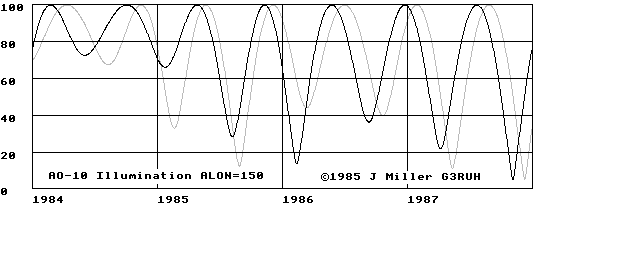Figure3. OSCAR-10 ILLUMINATION.  The effect of a +30 degree twist and the
nominal curve. In particular note the improvement in illumination obtained
around the beginning of 1985. However you can also see that the +30 degree
twist will be no good for the period of our example, Autumn 1985.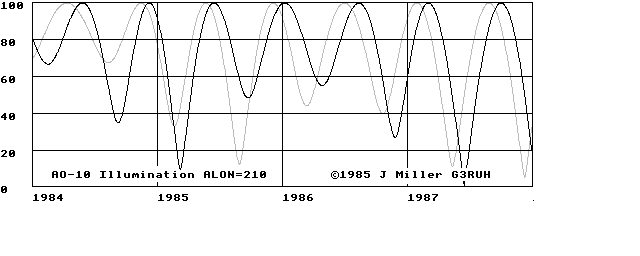Figure 4. OSCAR-10 ILLUMINATION showing the effect of a -30 degree adjustment
again superimposed on the nominal.  Now the null in August 1985 is raised
to 50%, still not too good, but better than the original of less than 20%.
A reorientation like this will have to be implemented around June 1st.

This would also mean peak activity now concentrated after apogee, in much
the same way as is presently done before it.  In addition, as I remarked
in O.N.49 page 3, eclipses are due in August 1985 around MA 85-130/256
when the transponder will have to be switched off.  All in all an

You might like to anticipate events of 1986-7, using the graphs.
Visualising these effects is made immeasurably easier  with my simple 3-D
model (O.N.44 et seq)  which  comprises  a hardboard  ellipse and small
globe.  It can be made in a couple of hours.  A table lamp a few metres
away can be used to represent the Sun, and casts a shadow nicely for
eclipse studies.  In part 3: ECLIPSES.

SUN'S UP!  Part 3

by J.R. Miller G3RUH

This is the final part of a series in which I've looked at the
relationship between the Sun and a satellite.  The first (O.N.50, December
1984) described a proper Sun model, whilst in the second (O.N.51, February
1985) vectors were explained and the results used to  investigate sun
angles and their effect on a satellite's solar illumination.

In this part I deal with eclipses.  Though I'm using Oscar-10 for my
examples the methods apply to any Earth satellite, low orbiters like
UOSAT, RS and NOAA through to OSCAR-10 and the geostationary comsats.

Satellite Eclipses
------------------
A satellite is said to be 'eclipsed' when it is within the Earth's shadow,
and the term 'shadowed' means the same thing.  You can visualise what is
happening in figure 5, which shows the shadow zone and a satellite within
it.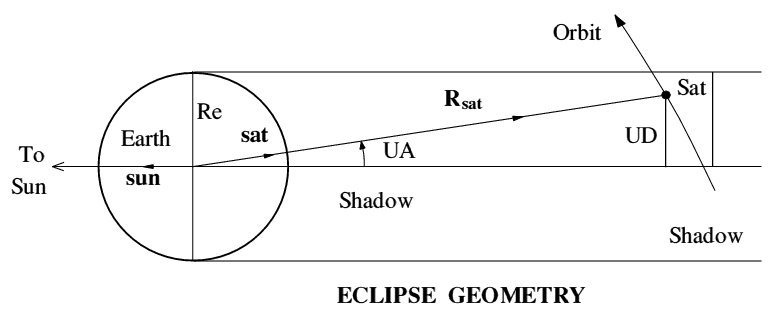Figure 5. Illustrating conditions of a satellite's solar eclipse.

It should be obvious that the only condition necessary for an eclipse is
that the distance marked UD (Umbral Distance) should be less than the
radius of the Earth, since this is the size of the shadow.

From the triangle, distance UD = Rsat * Sin(UA), so we need to work out
Rsat and the Umbral Angle UA.

Remembering the notes about vectors (pt. 2), the cosine of UA is simply
the scalar (dot) product of a unit vector AWAY from the Sun, and a unit
vector towards the satellite, i.e., denoting Cos(UA) by CUA:

CUA = - (Xsun*Xsat + Ysun*Ysat + Zsun*Zsat)

Since it's possible to get UD less than one Earth radius but on the SUNNY
side of Earth, we must also check that the satellite is on the dark side,
i.e. UA less than 90 degrees which means that CUA must be positive.

Sun and Satellite's Unit Position Vector
----------------------------------------
The Sun unit vector calculations were dealt with in part 1 of this series.

For the satellite we have to do the full orbital calculation, i.e. find
mean anomaly (MA), thence  eccentric  anomaly (EA) and the in-plane
coordinates (X and Y).  These are then rotated through argument of perigee
(AP), inclination (INCL) and then right ascension of the ascending node
(RAAN) until we arrive at the satellite's position vector in celestial
coordinates, the same as we used for the Sun.

First solve Kepler's equation (in radians) MA = EA - EC*Sin(EA) for EA
given  MA where EC  is  eccentricity.  This equation is 'transcendental'
which means it's a sod, and has to be resolved iteratively (see appendix).
Then for compactness in what follows denote:

CEA = Cos(EA):   SEA = Sin(EA)
CAP = Cos(AP):   SAP = Sin(AP)
CNC = Cos(INCL): SNC = Sin(INCL):     where INCL is Inclination.
CRA = Cos(RAAN): SRA = Sin(RAAN)

Rsat =           SMA*(1-EC*CEA):      where SMA = Semi Major Axis.
X =         (CEA-EC)/(1-EC*CEA):      Unit satellite vector in
Y = SQR(1-EC*EC)*SEA/(1-EC*CEA):      orbit plane.

Xsat = X*(CAP*CRA - SAP*CNC*SRA)  +  Y*( -SAP*CRA - CAP*CNC*SRA)
Ysat = X*(CAP*SRA + SAP*CNC*CRA)  +  Y*( -SAP*SRA + CAP*CNC*CRA)
Zsat = X*(SAP*SNC)                +  Y*(  CAP*SNC)

These  calculations are embodied in all elliptic orbit programs (they
use longitude of ascending node rather than RAAN because they work in
geocentric coordinates).  Equations above, though correct are inefficient
and in the appendix they are presented more nicely.

Eclipse - Worked Example
------------------------
Continuing the example started in parts 1&2, for Oscar-10 on 1985 August
12 at 0145 GMT.  If you flog through the arithmetic you will find: MA =
129.3, AP = 40.1, RAAN = 121.2 (all in degrees - see pt1. page 2).

Since EC = 0.61 we find EA = 147.9 degrees, and using a semi major axis of
SMA = 26100 km you get, in addition to a headache:

Xsat =  0.7864          Xsun = -0.7583 (as before. part 1 test data)
Ysat = -0.5923          Ysun =  0.5981
Zsat = -0.1755          Zsun =  0.2593

Rsat = 39582 km         CUA = 0.9961    UA = 5.09 deg
UD   =  3510 km

The Earth's (equatorial) radius is 6378 km and, since the umbral distance
is only 3510 km, at 0145 GMT August 12 1985 Oscar-10 will be eclipsed.

Observability
-------------
An MA of 129 degrees, (91/256 of the orbit) is 100 minutes before apogee,
and the event will be observable for an hour or so (on the telemetry) by
stations in Europe, Africa, the middle East and South America. The
transponder will not, of course, be switched on!

This 'eclipse season' lasts from 10th August to 3rd September 1985,and is
illustrated on page 3 of O.N.49, Oct 1984 with an eclipse diagram.

OSCAR-10 Planning Software
--------------------------
These ideas and others are embodied in an updated version of the program
which appeared as a pull-out centrefold in O.N. 45 (December 1983).
Written in BBC BASIC and called PLAN10 it's available from AMSAT-UK.
[ Updated 1990 Oct ].

It is well commented and serves as a useful comprehensive and
comprehensible set of reference equations for solving the elliptic orbit
problem, WITH DRAG.

It can be dissected and refashioned to individual needs.  It can be
translated into other BASIC dialects.

As coded, a sample PLAN10 output looks typically like the following,
though as is usual with BASIC programs, the time increment and most else
can be changed at will.  You will notice the data is the same as for
the examples in this series.

SATELLITE PREDICTIONS
---------------------
Location: G3RUH  Lat 52.208 Lon 0.059
Ephemeris Dated: 1985 Day 224

OSCAR-10  YEAR: 1985  ORBIT: 1627    ILL: 28%   SOLAR EL: 5 Deg

DAY:HHMM MA/256 MODE   RANGE     EL     AZ     SQ    UMD  ECL?
--------------------------------------------------------------
224:0100  75      B    34114     19    203     25   1.07    +
224:0115  80      B    35320     18    204     22   0.85   ECL
224:0130  86      B    36396     17    205     20   0.66   ECL
224:0145  91      B    37348     16    206     17   0.55   ECL
224:0200  97      B    38178     14    207     15   0.58   ECL
224:0215  102     B    38891     13    208     13   0.73   ECL
224:0230  108     B    39488     12    209     12   0.94   ECL
224:0245  113     B    39971     10    210     10   1.18    +
etc

Wither?
-------
If you have found this little series useful - or found it induced terminal
boredom, I should like to know.  I am quite happy to scribble more on the
numerical aspects of satellites - but you may not be interested!  Soon I
propose to write about drag, and maybe about the non-spherical Earth.
Perhaps you could suggest topics.  Please don't forget the SAE though.

[ I got 2 replies.  One said that the article was "mind-blowing", and
the other that I couldn't spell whither.  JRM ]

APPENDIX - Satellite Position Calculation
-----------------------------------------
Equations are given in BASIC, as they've been tested in that form.  By
separating out the coordinate transformations the number of multiplies and
additions are much reduced.  The  test  at  line  2140  is  not  set to
umpteen decimal  places.   Newton's  method is a 'second order' algorithm,
so as coded will converge to within 0.002*0.002/2 radians, (for Oscar-10,
this is equivalent to about 50 metres worst case position error, vastly
better than the ephemeris accuracy).  For definitions see part 1.

2000 REM Calculate Satellite Position
2010 REM ----------------------------
2020 REM Assumes mean anomaly (MA), Argument of perigee (AP) and Right
2030 REM Ascension of Ascending Node (RAAN) to have been calculated
2040 REM as well as inclination (INCL), eccentricity (EC), semi-major axis
2050 REM (SMA) and semi-minor axis (SMB). All angles in RADIANS.
2060
2070 REM Solve MA = EA - EC*SIN(EA) for EA given MA. (Newton's Method)
2080 EA = MA:                           REM Initial solution
2090 REPEAT
2100   C = COS(EA): S = SIN(EA)
2110   DNOM = 1-EC*C
2120   DE = (EA - EC*S - MA)/DNOM:      REM Change to EA for better
2130   EA =  EA - DE:                   REM  solution by this amount
2140 UNTIL ABS(DE) < 0.002:             REM  until converged. Coding as
2150                                    REM  fast as possible.
2160 Rsat = SMA*DNOM:                   REM Geocentric distance
2170
2180 REM Now compute satellite coordinates in plane of ellipse
2190 C  = COS(EA): S = SIN(EA)
2200 X1 = SMA*(C - EC)
2210 Y1 = SMB*S:                        REM Note: SMB=SMA*SQR(1-EC*EC)
2220 Z1 = 0
2230
2240 REM Rotate coordinates about Z through Argument of perigee
2250 C= COS(AP): S= SIN(AP)
2260 X2 = X1*C - Y1*S
2270 Y2 = X1*S + Y1*C
2280 Z2 = Z1
2290
2300 REM Rotate coordinates about X through Inclination
2310 C=COS(INCL):S=SIN(INCL)
2320 X3 = X2
2330 Y3 = Y2*C
2340 Z3 = Y2*S
2350
2360 REM Rotate about Z through RA of Ascending Node, and normalise
2370 C = COS(RAAN): S = SIN(RAAN):         REM with respect to Rsat
2380 Xsat = (X3*C - Y3*S)/Rsat
2390 Ysat = (X3*S + Y3*C)/Rsat
2400 Zsat = (Z3         )/Rsat
2410
2420 REM Satellite's position unit vector in celestial coordinates
2430 REM now calculated.  For geocentric coordinates use RAAN-GHAA
2440 REM above, instead of RAAN.

```

Feedback on these pages to Webmaster. Feedback on the article should be sent to James Miller

Created: 1994 Dec 04 -- Last modified: 2006 Feb 23